Wacker Art Clifford Algebra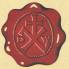Bild: "Edersee - Fähre Schein-Rehbach"

Prolog

"... geometry, you know, is the gate of science, and the gate is so low and small that one can only enter it as a little child."

William Kingdon Clifford

In mathematics the name Clifford Algebra is used but Clifford himself named his algebra Geometric Algebra. For an Overview on the subject visit my Geometric Algebra page. On this page it is assumed that the reader is familiar with the basic concepts of geometric algebra.

Clifford Algebra

The Inner Product

We start with the geometric product of the two vectors u and v: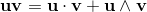Exchanging the vectors u and v gives the relation: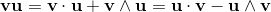By adding the two equations we get the scalar product expressed with the help of the geometric product:Or in a more general form:Building the square of a vector with the geometic product we get:Which is the scalar product of the vector. That means we get the square length of the vector.

The relation of geometric products of the ortogonormal basic vectors, can be written with the help of geometic products as follows:Or in a more general form with the metric tensor when the base vectors are not orthogonal.If we square the sum of two vectors (using the geometric product) we get:(1)

Reordering the elements gives:(2)

If we square the difference of two vectors we get:(3)

Reordering the elements gives: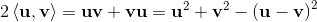(4)

Summing up equation (2) and (4) gives equation (5):(5)

We now have the following relations for the scalar product of two vectors:(2)(4)(5)

The Outer Product

The outer product can be defined in dependence from the geometric product as follows: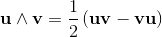Using the following product relation:(6)

Gives the following relation for the outer product: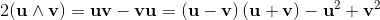(7)

Using the following product relation: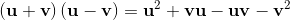(8)

Gives a further relation for the outer product:(9)

The diverens between the given two relations for the outer product results in the folowing expression: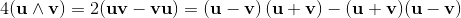(10)

We now have the following relations for the outer product of two vectors:Geometric Product

When we sum up the results for the inner (5) and the outer product (10) we come back to the geometric product: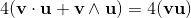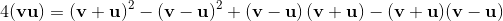Geometric Algebra and Lie-Algebra (Commutator Symbol)

Commutator Symbol

The commutator symbol is defined as [a,b] = ab-ba.

If we apply the commutator symbol on vectors a, b with the geometric product as operation, we get the following expression for the outer product:

2ab = [a, b] = ab-ba;

2ab = [a, b] = -[b, a] = -(ba-ab) = -2ba;

2aa = [a,a] = aa - aa = 0;

λ[a, b] = [λa, b] = λ(ab - ba) = [a, λb];

[a+b,c] = [a,c] + [b,c] = (a+b)c - c(a+b) = ac + bc - ca - cb = (ac - ca) + (bc - cb);

[a, [b, c]] = a(bc - cb) - (bc - cb)a = abc - acb - bca + cba;

[[a, b], c] = (ab - ba)c - c(ab - ba) = abc - bac - cab + cba;

Jacobi Identity:

[a, [b, c]] + [b, [c, a]] + [c, [a, b]] = 0;

[a, bc - cb] + [b, ca - ac] + [c, ab - ba] = 0;

(abc - acb) - (bca - cba) + (bca - bac) - (cab - acb) + (cab - cba) - (abc - bac) = 0;

abc - abc + acb - acb + bca - bca + cba - cba + bac - bac + cab - cab = 0;

Anticommutator Symbol

2ab = {a, b} = ab + ba;

The Geometric Product with Commutator and Anticommutator Symbol

2ab = 2ab + 2ab = {a, b} + [a, b] = ab + ba + ab - ba;

Motivation for a Lie-Algebra

With a matrix A we can define a element a = (IA) and a second element b = (IB) from a matrix B, both close to the identity element I for small ε.

The product will be:

ab = (IA)(IB) = I + ε(A+B) +ε2AB;

If we ingnore the AB term because ε2 is very small we get:

ab = I + ε(A+B);

With a-1 = (IA)-1 = I - εA + ε2A2 - ε3A3... ;

For non commutating elements a,b the expression aba-1b-1 is not equal I. We can express this term as follows:

aba-1b-1 = (IA)(IB)(I - εA + ε2A2)(I - εB + ε2B2);

Suppressing terms with εn and n>2 will give:

aba-1b-1 = I + ε2(AB - BA).

Definiton of a Lie Group can be found on the Abstract Algebra page:

Bild: "Edersee - Weißer Stein"

Vector Bivector Products I

The following example of a vector and a bivector product can be generalised to arbitary vectors.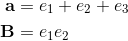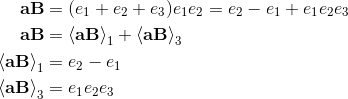The result of the product is a vector part and a trivector part.The result of the product is a vector part and a trivector part.For the example we get the following result that can be generalised: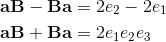The difference separates the vector part and the sum separates the trivector part.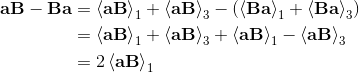We can know define the inner and the outer product for a vector with a bivector.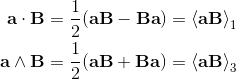The scalare product of a vector with a bivector is anticommutative and the outer product is commutative.Now we can write down the general form of the product of a vector with a bivector: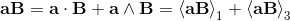Vector Bivector Products II

Double Products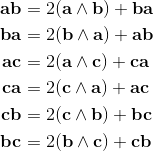Trible Products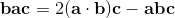The Vector Bivector Products(1)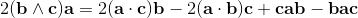(2)If we build the difference between (1) and (2) we come to the following expression: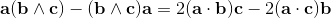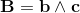With the letter B for the bivector (bc) it is possible to rewrite this equation in the following form.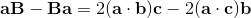On the right side is a Vector quantity. That means this operation is grade lowering.

The grade lowering property of the expression is used to define the scalare product of a vector with a bivector.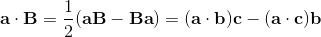This definition will result in the following relation: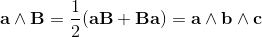Vector - Bivector RelationsFrom this relation we get:Renaming the vector b to b1. And then multiplying from the right with the vector b2 gives:Decomposing the third term in the same manner as before, results in the following expression: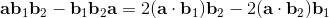From this equations we come to the following general formular for the inner product of a vector with a blade:The bi with the inverted circumflex is omitted from the product.

Bild: "Edersee bei Niedrigwasser - Fürstental"

The Bivector Square

Preparation

Basic relations used in the deduction of the formular of the bivector square.

Expressing the Wedge Product by the Scalare Product and the Geometric ProductInsert in (1)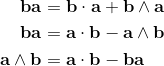Insert in (1)

Pythagorean Relation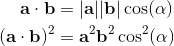Insert in (7)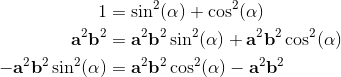Insert in (8)

Deduction of the basic relations of the Bivector Square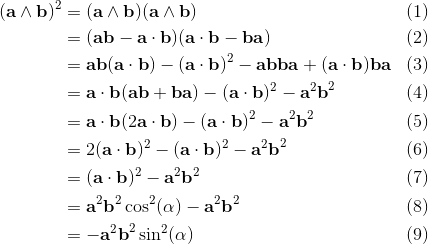The next page is about Geometric Algebra and Matrices.

27. August 2017 Version 1.0
Copyright: Hermann Wacker Uhlandstraße 10 D-85386 Eching bei Freising Germany Haftungsausschluß